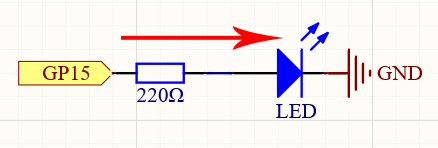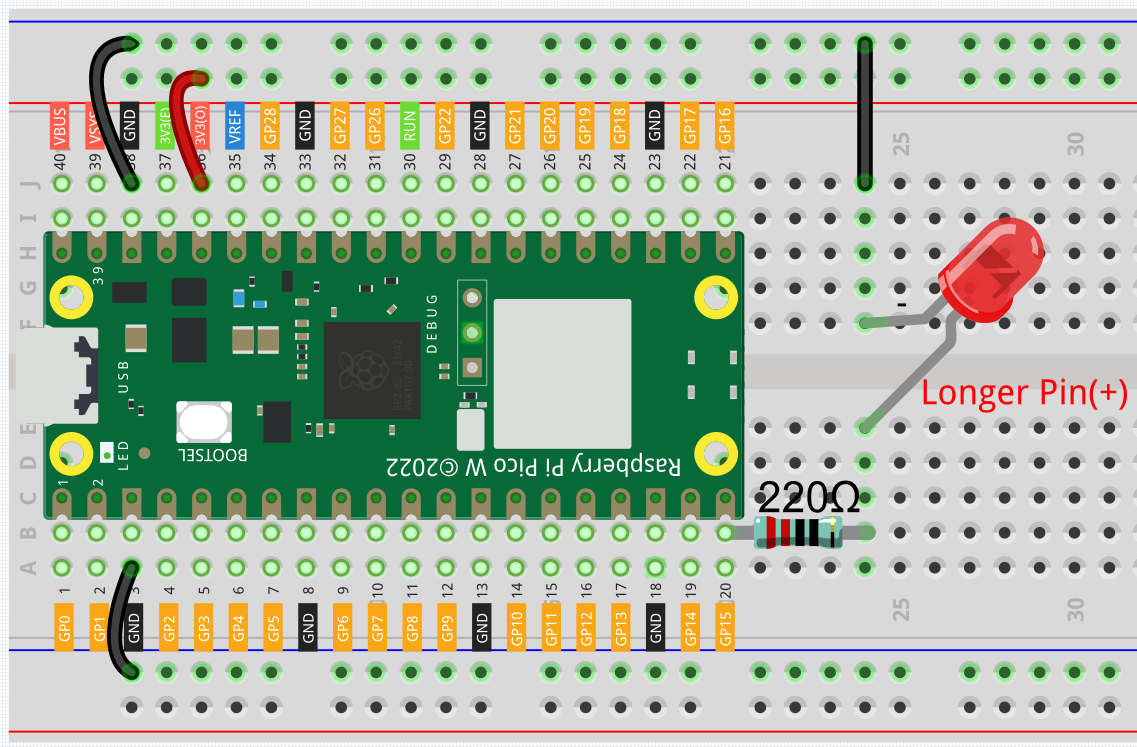# 2.1 - Hello, LED!¶

Just as printing “Hello, world!” is the first step in learning to program, using a program to drive an LED is the traditional introduction to learning physical programming.

Required Components

In this project, we need the following components.

Name

ITEMS IN THIS KIT

Kepler Kit

450+

Kepler Kit

SN

COMPONENT INTRODUCTION

QUANTITY

1

Raspberry Pi Pico W

1

2

Micro USB Cable

1

3

1

4

Jumper Wires

Several

5

Resistor

1(220Ω)

6

LED

1

SchematicThe principle of this circuit is simple and the current direction is shown in the figure. When GP15 outputs high level(3.3v), the LED will light up after the 220ohm current limiting resistor. When GP15 outputs low level (0v), the LED will turn off.

WiringLet us follow the direction of the current to build the circuit!

1. Here we use the electrical signal from the GP15 pin of the Pico W board to make the LED work, and the circuit starts from here.

2. The current needs to pass through a 220 ohm resistor (used to protect the LED). Insert one end (either end) of the resistor into the same row as the Pico W GP15 pin (row 20 in my circuit), and insert the other end into the free row of the breadboard (row 24 in my circuit).

3. Pick up the LED, you will see that one of its leads is longer than the other. Insert the longer lead into the same row as the end of the resistor, and connect the shorter lead across the middle gap of the breadboard to the same row.

4. Insert the male-to-male (M2M) jumper wire into the same row as the LED short pin, and then connect it to the negative power bus of the breadboard.

5. Use a jumper to connect the negative power bus to the GND pin of Pico W.

Code

Note

• You can open the file `2.1_hello_led.ino` under the path of `kepler-kit-main/arduino/2.1_hello_led`.

• Or copy this code into Arduino IDE.

• Don’t forget to select the board(Raspberry Pi Pico) and the correct port before clicking the Upload button.

After the code runs, you will see the LED blinking.

How it works?

Here, we connect the LED to the digital pin 15, so we need to declare an int variable called ledpin at the beginning of the program and assign a value of 15.

```const int ledPin = 15;
```

Now, initialize the pin in the `setup()` function, where you need to initialize the pin to `OUTPUT` mode.

```void setup() {
pinMode(ledPin, OUTPUT);
}
```

In `loop()`, `digitalWrite()` is used to provide 3.3V high level signal for ledpin, which will cause voltage difference between LED pins and light LED up.

```digitalWrite(ledPin, HIGH);
```

If the level signal is changed to LOW, the ledPin’s signal will be returned to 0 V to turn LED off.

```digitalWrite(ledPin, LOW);
```

An interval between on and off is required to allow people to see the change, so we use a `delay(1000)` code to let the controller do nothing for 1000 ms.

```delay(1000);
```